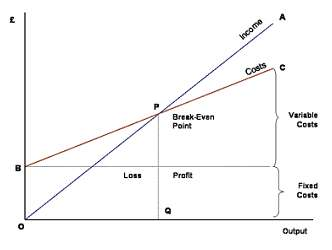# Break Even Point

## What is Break Even Point?

Break even point (BEP) is the point where the profit from the transaction is zero and the total sales is equal to total costs. Break even point is he inflection point where the revenue sales are same as the costs. At the break even point, there is zero profit or zero loss for the company.

Break even point is important for companies to understand the minimum business required to sustain any product or service. The units break even i.e. number of units to be sold at the break even pricing helps in evaluating the break even point. This method of evaluation is known as break even analysis.

## Formula for Break Even Point

Break even point (BEP) = Fixed Costs/(Selling Price – Variable Costs)

Fixed Costs: These are the costs that do not change with the quantity produced and remains constant. Examples can be the rent, property tax, insurance, etc.

Variable Costs: These costs depend on the quantity produced and vary with the production levels. They increase with the increase in production volumes.Examples are labor cost, material cost, etc.

Total Cost = Fixed Cost + Variable Cost

Break Even Chart: It is a graphical representation of costs at different levels as shown below-P represents the breakeven point where the income is equal to the total costs and the profit is zero.

## Importance of Break Even Point

Break even point is an important aspect for any business. It gives that inflection point for any business for understanding the profitability for a company. The break even point is calculated as the ratio between costs and revenues. The various scenarios for calculating the break even point are:

1. If break even point is less than 1: This means that the costs are lower than the revenues & hence the company is profitable

2. If break even point is 1 : This means that the costs & revenues are equal, i.e. there is not profit or loss

3. If break even point is greater than 1: This means that the costs are higher than the revenues, i.e. the company is loss making

This article has been researched & authored by the Business Concepts Team. It has been reviewed & published by the MBA Skool Team. The content on MBA Skool has been created for educational & academic purpose only.

Browse the definition and meaning of more similar terms. The Management Dictionary covers over 2000 business concepts from 6 categories.

Search & Explore : Business Concepts